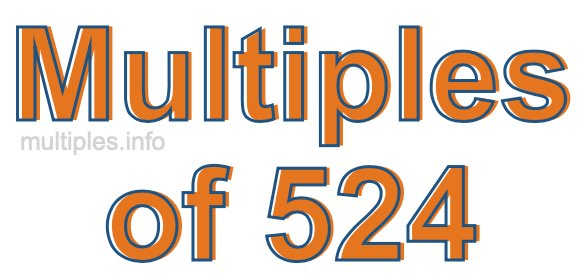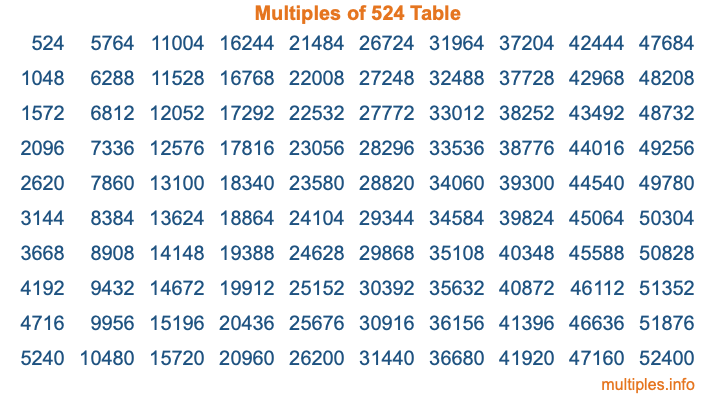Multiples of 524Welcome to the Multiples of 524 page. Here we will first teach you everything you will ever need to know about the multiples of 524, and then give you a study guide summary of everything we taught you to make sure you remember it all. Use this page to look up facts and learn information about the multiples of 524. This page will make you a multiples of five hundred twenty-four expert!

Definition of Multiples of 524
Multiples of 524 are all the numbers that when divided by 524 equal an integer. Each of the multiples of 524 are called a multiple. A multiple of 524 is created by multiplying 524 by an integer.

Therefore, to create a list of multiples of 524, you start with 1 multiplied by 524, then 2 multiplied by 524, then 3 multiplied by 524, and so on for as long as you want. Thus, the list of the first five multiples of 524 is 524, 1048, 1572, 2096, and 2620. To see a larger list of multiples of 524, see the printable image of Multiples of 524 further down on this page. We also have a category where you can choose any nth multiple of 524.

Multiples of 524 Checker
The Multiples of 524 Checker below checks to see if any number of your choice is a multiple of 524. In other words, it checks to see if there is any number (integer) that when multiplied by 524 will equal your number. To do that, we divide your number by 524. If the the quotient is an integer, then your number is a multiple of 524.

Is  a multiple of 524?

Least Common Multiple of 524 and ...
A Least Common Multiple (LCM) is the lowest multiple that two or more numbers have in common. This is also called the smallest common multiple or lowest common multiple and is useful to know when you are adding our subtracting fractions. Enter one or more numbers below (524 is already entered) to find the LCM.

Check out our LCM Calculator if you need more details about the Least Common Multiple or if you need the LCM for different numbers for adding and subtraction fractions.

nth Multiple of 524
As we stated above, 524 is the first multiple of 524, 1048 is the second multiple of 524, 1572 is the third multiple of 524, and so on. Enter a number below to find the nth multiple of 524.

th multiple of 524

Multiples of 524 vs Factors of 524
524 is a multiple of 524 and a factor of 524, but that is where the similarities end. All postive multiples of 524 are 524 or greater than 524. All positive factors of 524 are 524 or less than 524.

Below is the beginning list of multiples of 524 and the factors of 524 so you can compare:

Multiples of 524: 524, 1048, 1572, 2096, 2620, etc.

Factors of 524: 1, 2, 4, 131, 262, 524

As you can see, the multiples of 524 are all the numbers that you can divide by 524 to get a whole number. The factors of 524, on the other hand, are all the whole numbers that you can multiply by another whole number to get 524.

It's also interesting to note that if a number (x) is a factor of 524, then 524 will also be a multiple of that number (x).

Multiples of 524 vs Divisors of 524
The divisors of 524 are all the integers that 524 can be divided by evenly. Below is a list of the divisors of 524.

Divisors of 524: 1, 2, 4, 131, 262, 524

The interesting thing to note here is that if you take any multiple of 524 and divide it by a divisor of 524, you will see that the quotient is an integer.

Multiples of 524 Table
Below is an image of the first 100 multiples of 524 in a table. The table is in chronological order, column by column. The first column has the first ten multiples of 524, the second column has the next ten multiples of 524, and so on.The Multiples of 524 Table is also referred to as the 524 Times Table or Times Table of 524. You are welcome to print out our table for your studies.

Negative Multiples of 524
Although not often discussed or needed in math, it is worth mentioning that you can make a list of negative multiples of 524 by multiplying 524 by -1, then by -2, then by -3, and so on, to get the following list of negative multiples of 524:

-524, -1048, -1572, -2096, -2620, etc.

Multiples of 524 Summary
Below is a summary of important Multiples of 524 facts that we have discussed on this page. To retain the knowledge on this page, we recommend that you read through the summary and explain to yourself or a study partner why they hold true.

There are an infinite number of multiples of 524.

A multiple of 524 divided by 524 will equal a whole number.

524 divided by a factor of 524 equals a divisor of 524.

The nth multiple of 524 is n times 524.

The largest factor of 524 is equal to the first positive multiple of 524.

524 is a multiple of every factor of 524.

524 is a multiple of 524.

A multiple of 524 divided by a divisor of 524 equals an integer.

524 divided by a divisor of 524 equals a factor of 524.

Any integer times 524 will equal a multiple of 524.

Multiples of a Number
Here you can get the multiples of another number, all with the same attention to detail as we did for multiples of 524 on this page.

Multiples of
Multiples of 525
Did you find our page about multiples of five hundred twenty-four educational? Do you want more knowledge? Check out the multiples of the next number on our list!